# Calculate the residual stress on a beam after bending

freddie_mclair
TL;DR Summary
After being a beam is bent, due to the springback effect, the resulting bent radius is larger than the bending radius. How can I estimate the residual stress that remains in the beam after an elasto-plastic loading/bending?
Hello everyone, I hope you can give me hand with what I will present.

I have a beam with cross-section shown below.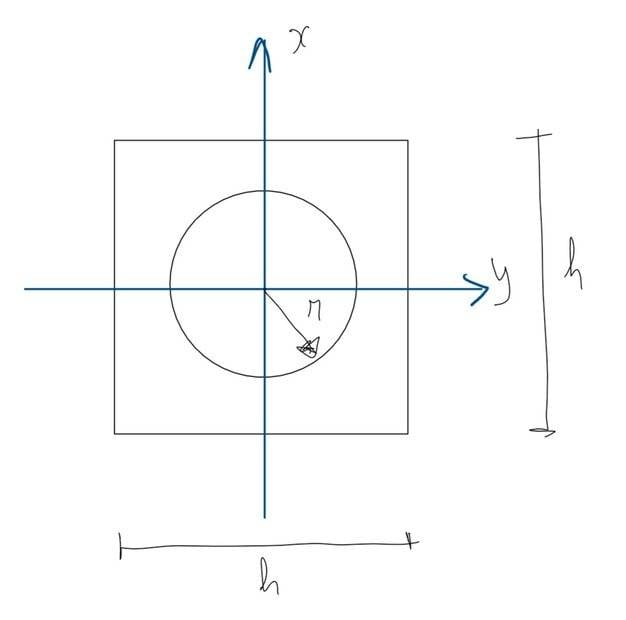I considered a simple linear hardening model for predicting the stress and strain developed by bending the beam on the radius ##R_0## around the y-axis, where:

The strain, ##\varepsilon_L(x)##, can be defined as:
##\varepsilon_L(x) = \frac{x}{R_0}##

And the stress, ##\sigma_L(x)##, developed in the beam due to the loading by bending is:
##\sigma_L(x) = \begin{cases}
\frac{x}{R_0} \cdot E \mbox{, if } x \leq \varepsilon_y \cdot R_0\\
\sigma_L(x) = S_y+(\frac{x}{R_0}-\varepsilon_y)\cdot E_1\mbox{, if } x \gt \varepsilon_y \cdot R_0
\end{cases}##

Where and ##\varepsilon_y = \frac{S_y}{E}##, ##S_y## is the yield strength and ##E## the Young's Modulus.
##E_1## is the tangent modulus which is defined as shown in the picture below.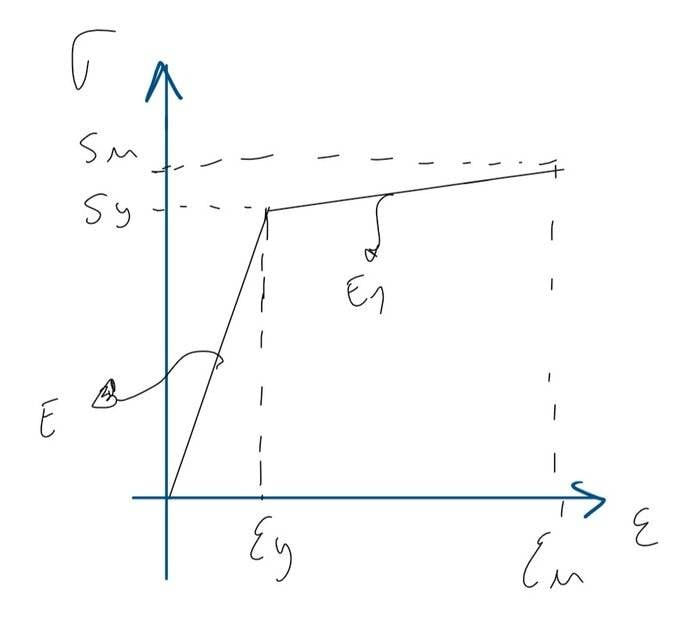I also know that, due to the springback effect, the relationship between the bending radius ##R_0## and the resulting radius ##R_r## is given by:
##\frac{1}{R_0} - \frac{1}{R_r} = \frac{M_b}{EJ}##

Where ##M_b## is the bending moment and ##J## the moment of inertia (in this case ##J=\frac{h^4}{12}-\pi \frac{r^4}{4}##).

The bending moment can be estimated by:
##M_b=2\int_0^{h/2} \sigma_L(x) b(x) x dx##

Where ##b(x)\cdot x## is the cross-sectional area of the beam and ##b(x)## is defined by:
##b(x) = \begin{cases}
h-2\sqrt{r^2-x^2} \mbox{, if } x \leq r\\
h \mbox{, if } x \gt r
\end{cases}##

Now, with all this at hand, how can I estimate the residual stress on the beam after bending, i.e., after unloading the beam?

Dr.D
Work through the process that the beam goes through. Calculate the part of the material that has been yielded, and then allow the beam to relax. As it relaxes, there will be internal stresses locked up inside that come to an equilibrium condition. It is a lengthy problem, but should not be insurmountable if you really want to do it.

•Lnewqban
FEAnalyst
Indeed, elasto-plastic bending with hardening accounted for and unusual cross-section is not an easy thing to solve by hand. In books you will find derivations and examples mostly for rectangular beams and perfect plasticity. But you should be able to modify them for your case (books often provide some hints about how to proceed with other types of beams and more advanced material models). For verification you can use Finite Element Analysis (in open-source software if you don’t have a license for a program like that). FEA will show you everything for every possible scenario - you can get stress and strain distributions (including equivalent plastic strain), displacements and so on. Of course it’s not a problem to unload the beam in FEA or even load it several times to see how various complex material behaviors influence the results.

freddie_mclair
Thanks guys for your reply, but I'm looking for an analytical solution. The first thing I did was to compute what is the necessary bending moment, ##M_b## to get the radius I need, ##R_k## by computing the integral in Mathematica.
Then, with ##M_b## I can also get the stress profile in the cross-section by using ##\sigma_b = \frac{M_b}{J}x##.
But then, what do I have to subtract from ##\sigma_b## to get the residual stress after unloading?

freddie_mclair
Ah! I had an enlightenment moment! (I think!)

What I calculated, ##M_b##, is in fact the bending moment accounting the springback effect, i.e., taking into account the release of the stored energy. This means that, ##\sigma_b## will give me the stress in the beam after unloading, right?
Therefore, the residual stress, ##\sigma_{Res}##, will be just the difference between ##\sigma_L## and ##\sigma_b##:
##\sigma_{Res}(x) = \sigma_L(x) - \sigma_b(x)##

I made a quick plot in Excel, and I think the results look good (at least compliant with the literature).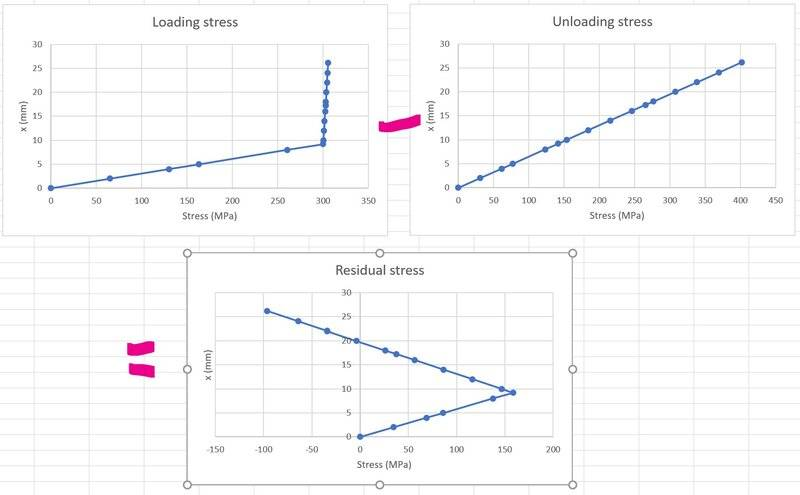FEAnalyst
Here's an exemplary stress-strain curve obtained from FEA of a simply-supported rectangular beam subjected to uniformly distributed load (the material model was bilinear with hardening):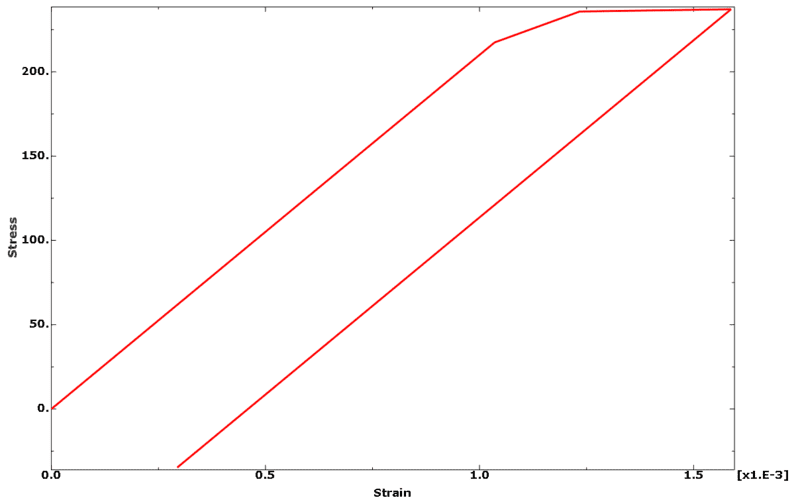I was referring to using FEA just to verify your analytical calculations.

Can you share the titles of books that you use for these calculations ? I'm also interested in similar topics and maybe I'll get to know new interesting literature.

Dr.D
@FEAnalyst : That's a strange loading diagram in that the stress rises instantaneously at zero strain. I don't know of any materials like that. I don't think I'd call it exemplary.

Also, there is nothing in FEA that can create a stress-strain curve, other than what the code writers built into the software.

freddie_mclair
@FEAnalyst I haven't used any particular book, I got this elasto-plastic formulation from browsing the web.

I started from simple assumptions, like a bilinear elasto-plastic stress-strain "curve" as the one I defined in the first post. Then, a simple linear hardening model to match it:

##\sigma(\varepsilon) = \begin{cases}
\varepsilon E \mbox{, if } \varepsilon \leq \varepsilon_y\\
S_y + (\varepsilon - \varepsilon_y)\cdot E_1 \mbox{, if } \varepsilon \gt \varepsilon_y
\end{cases}##

I have defined ##E_1## to be ##E_1 = 0.01 E##.

Then, I found somewhere a nice relationship (which should have been obvious) between the bending radius and the strain developed in the beam, allowing you to switch from the strain and define the stress in terms of the beam dimensions:

##\varepsilon_L(x) = \frac{x}{R_0}##

Which then results in the formulation I have also defined in the first post:

##\sigma_L(x) = \begin{cases}
\frac{x}{R_0} \cdot E \mbox{, if } x \leq \varepsilon_y \cdot R_0\\
\sigma_L(x) = S_y+(\frac{x}{R_0}-\varepsilon_y)\cdot E_1\mbox{, if } x \gt \varepsilon_y \cdot R_0
\end{cases}##

I have found the sprinback equation on the web, and then, using several other websites I managed to derive it myself as described in the attached PDF. Please, take this with a pinch of salt because it might have some errors on the wording and maybe also on the mathematical formulation I have defined there.

So, the springback equation is also in my first post:

##\frac{1}{R_0} - \frac{1}{R_r} = \frac{M_b}{EJ}##

Then, what I did was the following:
1. Computed the required bending moment using the integral ##M_b=2\int_0^{h/2} \sigma_L(x) b(x) x dx##, to get my desired radius after springback, ##R_r##, and taking care of the different elastic and plastic regions;
2. Computed the stress profile, ##\sigma_b(x)##, due to the bending moment ##M_b##, which in fact corresponds to the unloading stress (after springback) by simply doing: ##\sigma_b(x) = \frac{M_b}{J}x##;
3. Using the loading curve defined by ##\sigma_L(x)##, I managed to create the stress profile for ##\sigma_L(x)##;
4. The residual stress profile, ##\sigma_{Res}(x)## is then computed by doing ##\sigma_{Res}(x)= \sigma_L(x) - \sigma_b(x)##.
The hardest part was to calculate ##M_b## due to the strange geometry I have, which led to non-trivial integrals. But Mathematica helped me with them.

The stress profiles for ##\sigma_L(x)## and ##\sigma_b(x)## are in my previous post. But please be aware that they are not stress-strain curves! They are defined in terms of the beam cross-section geometry.

Hope this helps!

#### Attachments

• master.pdf
214 KB · Views: 121
Last edited:
FEAnalyst
That's a strange loading diagram in that the stress rises instantaneously at zero strain. I don't know of any materials like that. I don't think I'd call it exemplary.
Could you attach a simple sketch showing how this should look like ? Maybe something must be changed in the approach to this simulation. Load was ramped (uniformly increasing) in the first step of the analysis. Stress-strain curve was obtained by combining the total normal strain in the direction of beam axis with normal stress in the same direction. Both stress and strain were read from the bottom most layer of elements in the middle of the beam and averaged.

@freddie_mclair Thank you for sharing your results.

Dr.D
Assuming no residual stress at the beginning, the stress-strain curve should pass through (0,0). There is no physical mechanism by which it can then jump to a positive stress value with no strain.

FEAnalyst
Assuming no residual stress at the beginning, the stress-strain curve should pass through (0,0). There is no physical mechanism by which it can then jump to a positive stress value with no strain.

But it starts from (0,0):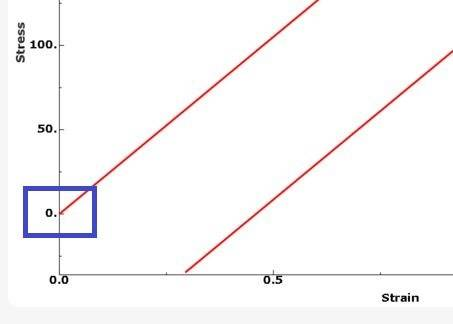Dr.D
You are correct about it starting from (0,0); I failed to see that. I assumed that the horizontal axis line was at zero stress; that's the way I've always seen it plotted.

This raises the question, however, about what does it mean at the other end where the stress goes negative?

FEAnalyst
I was also wondering why the stress changes sign at the end of unloading.

Here's what happens with normal stress in the middle part of the beam at each frame during unloading (contour plot is synchronized with stress-strain curve and the dark blue dot shows the current location on the curve):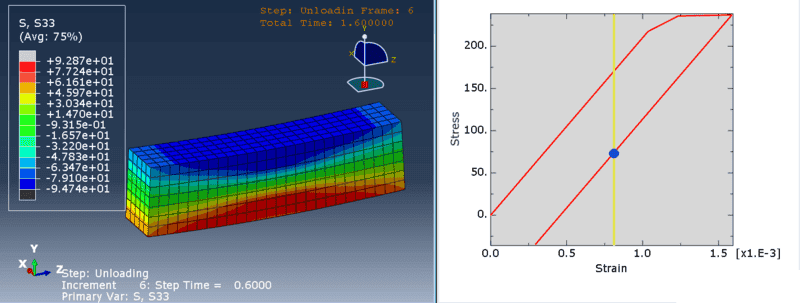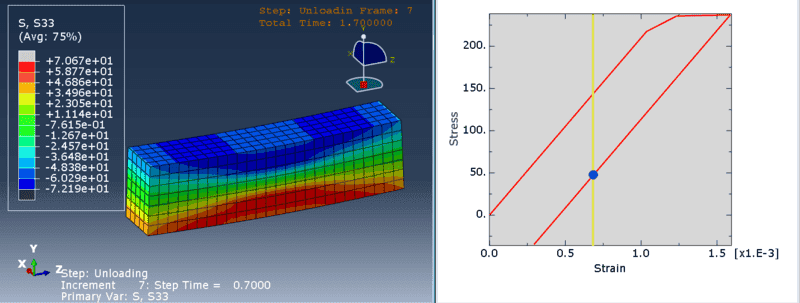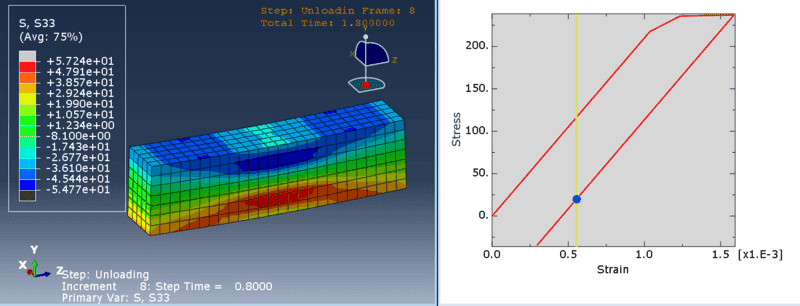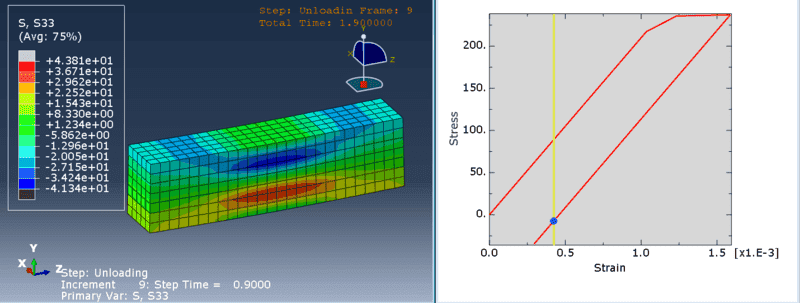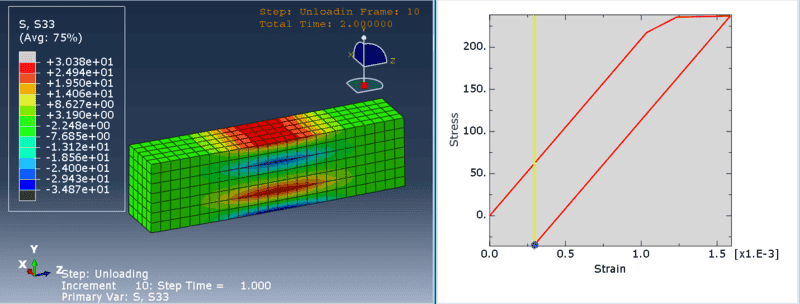In the last two frames stress has different sign. Maybe those pictures will let you interpret the behavior of this model.

P.S. Unloading analysis step was realized in such a way that the load was removed gradually throughout this step allowing the beam to regain its shape (at least partially since there's also some permanent deformation).

•PhDeezNutz
onatirec
the negative stress appears to be the residual stress after relaxation. Seems you are plotting not the middle of the bar (which would be right along the neutral axis) but perhaps the middle of the the lower surface; it's put in tension during the bending, would be in slight compression after unloading.

I'm curious as to how we are defining "residual stress on a beam" - peak?. I would suspect that the total residual stress state would be geometrically distributed and would want to find an equilibrium (obviously this isn't mathematically precise); there would be contributions from both tension and compression as seen above.

FEAnalyst
the negative stress appears to be the residual stress after relaxation. Seems you are plotting not the middle of the bar (which would be right along the neutral axis) but perhaps the middle of the the lower surface; it's put in tension during the bending, would be in slight compression after unloading.
Yes, I took the stresses from elements in the bottom most layer and averaged them. And when I was talking about the middle of the beam, I actually meant the middle between the supports (in the beam's longitudinal axis).

onatirec
...bottom most layer ... middle between the supports (in the beam's longitudinal axis).

that makes sense

Mentor
This diagram, from Metal Forming - Mechanics and Metallurgy by Hosford and Caddell, shows how to calculate residual stresses after plastic bending and force removal for a simple elastic-plastic material: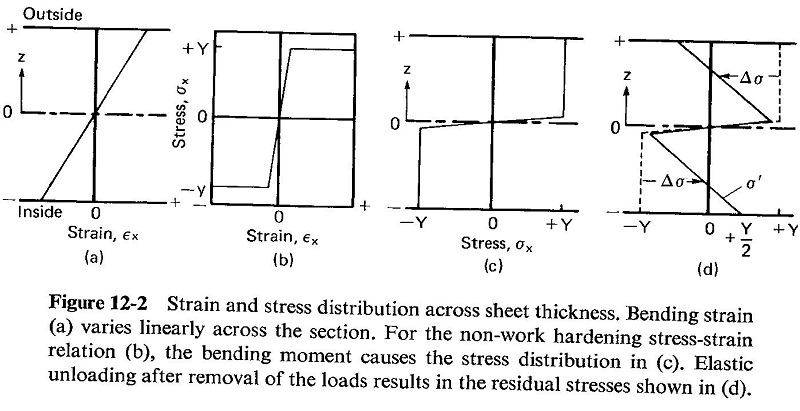It is used in springs to reduce peak surface tensile stress under load. Search term preset (or presetting) springs to learn more.

A basic calculation in sheet metal forming is to calculate the amount of springback when bending sheet metal. The above diagram is for simple bending. The calculation is even more fun when calculating bending under tension for the purpose of reducing springback.

freddie_mclair
I was also wondering why the stress changes sign at the end of unloading.

Here's what happens with normal stress in the middle part of the beam at each frame during unloading (contour plot is synchronized with stress-strain curve and the dark blue dot shows the current location on the curve):

View attachment 287860

View attachment 287861

View attachment 287862

View attachment 287863

View attachment 287864

In the last two frames stress has different sign. Maybe those pictures will let you interpret the behavior of this model.

P.S. Unloading analysis step was realized in such a way that the load was removed gradually throughout this step allowing the beam to regain its shape (at least partially since there's also some permanent deformation).
Thanks a lot for sharing this.
This is very interesting! Which software are you using?

FEAnalyst
Thanks a lot for sharing this.
This is very interesting! Which software are you using?
I work in Abaqus. It's extremely powerful when it comes to nonlinear solid mechanics. Let me know if you would like to solve something with FEA, I will be glad to help you with that.

freddie_mclair
Hi! Thanks a lot!
I think it could be very interesting if you could model with FEA what I have modeled analytically on my first post.

The cross-section geometry is the one I defined in my first post: it's a squared section with ##h=51.9\mbox{ mm}## and a circular hole with a radius of ##r=17.65\mbox{ mm}##. The bending radius (before springback) is ##R_0=4917.65\mbox{ mm}##; from my analytical model, after springback, I get a radius of ##R_k=11707.5mm##.

The peak stresses I get are the following: ##\sigma_{res1}=190\mbox{ MPa}## at the elastic-plastic core boundary and ##\sigma_{res2}=-140\mbox{ MPa}## at the outer surface of the beam. Here's the plot I get (I only plotted half of the beam, the other part is symmetric):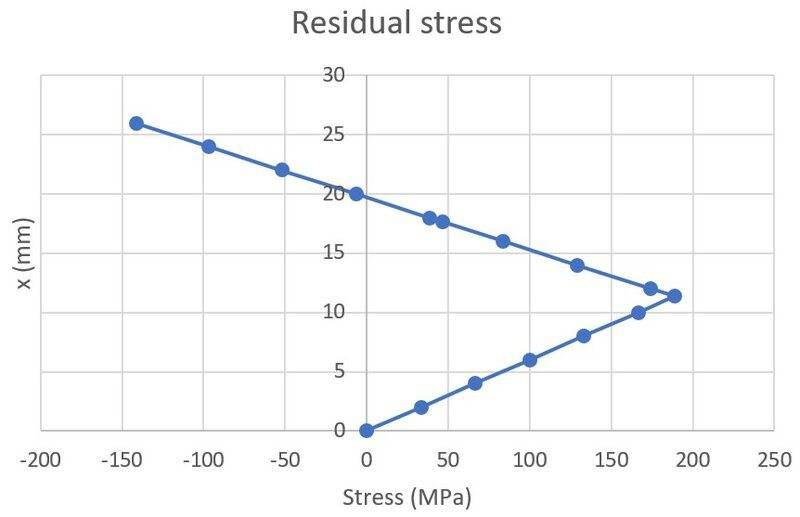Last edited:
freddie_mclair
Hi! Thanks a lot!
I think it could be very interesting if you could model with FEA what I have modeled analytically on my first post.

The cross-section geometry is the one I defined in my first post: it's a squared section with ##h=51.9\mbox{ mm}## and a circular hole with a radius of ##r=17.65\mbox{ mm}##. The bending radius (before springback) is ##R_0=4917.65\mbox{ mm}##; from my analytical model, after springback, I get a radius of ##R_k=11707.5mm##.

The peak stresses I get are the following: ##\sigma_{res1}=190\mbox{ MPa}## at the elastic-plastic core boundary and ##\sigma_{res2}=-140\mbox{ MPa}## at the outer surface of the beam. Here's the plot I get (I only plotted half of the beam, the other part is symmetric):

View attachment 287995
Sorry, I forgot to mention the mechanical properties of the material (SS 306LN):
- Yield strength: ##S_y = 450\mbox{ MPa}##
- Young's modulus: ##E = 190\mbox{ GPa}##
- Tangent modulus: ##E_1 = 0.01\cdot E##

FEAnalyst
To model this I would also need to know what are the boundary conditions (simply-supported beam ? cantilever ?), length of the beam and loading (type and magnitude).

And one more thing - ultimate tensile strength with elongation at failure, if you have it.

That should suffice.

Last edited:
freddie_mclair
To model this I would also need to know what are the boundary conditions (simply-supported beam ? cantilever ?), length of the beam and loading (type and magnitude).

And one more thing - ultimate tensile strength with elongation at failure, if you have it.

That should suffice.
Hello!

Sorry for the delay on my reply!

I don't know the tensile strength and elongation at failure.

For the load, I'm not sure the best way to define it. My model is independent of the load. I was able to calculate the total bending moment by just considering the required bending radius. The total moment I get is:

##M_b=12 \mbox{ kN m}##.

But, I'm not sure how to translate this bending moment to a load... should we just consider a point load in the middle of the beam? If we do this, then the load would be ##P=4M_b/l##, where ##l## is the length.

Now, for the setup, I would use a simply supported beam. For the length, I don't know, mainly because my model is length-independent. I have only checked the cross-sectional stress distribution. I think a 1meter beam should be OK for simulating the results.

What do you think?

Thanks!

freddie_mclair
Hello everyone,

I have finally modeled a bilinear elasto-plastic bending phenomena in ANSYS in order to cross-check my analytical calculation.
By applying the bending moment I defined in the post above on a straight beam, and taking also all the mechanical and geometric properties I have defined in the previous post, I can say that the FEM values match very well the theoretical ones!

From the analytical model, the residual stress profile across the cross-section of the beam is the following: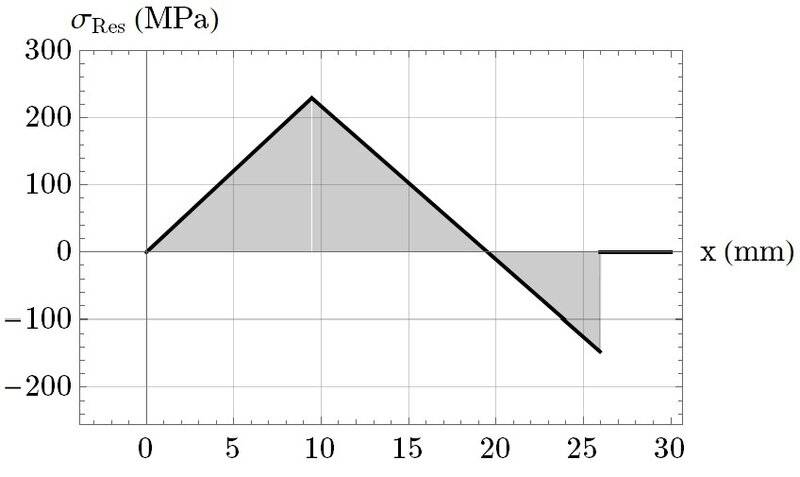From the ANSYS model, after applying the bending moment, the beam gets indeed bent, and after springback, I got the following shape and normal stress distribution in the zz direction: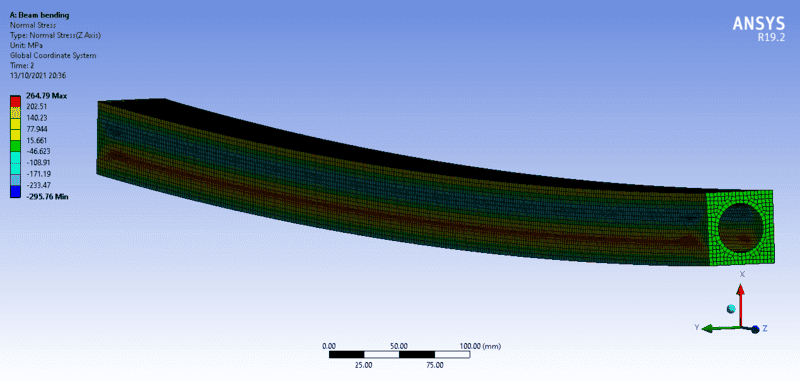The cross-sectional residual stress distribution (in the middle of the beam) is: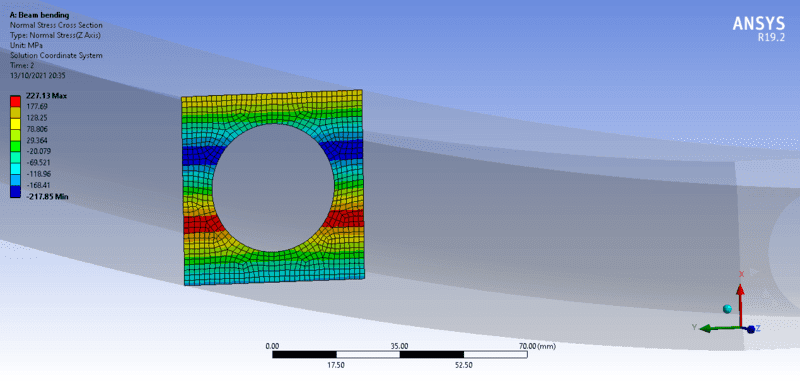Then, finally, plotting the residual stress profile across a path of this cross-section I get: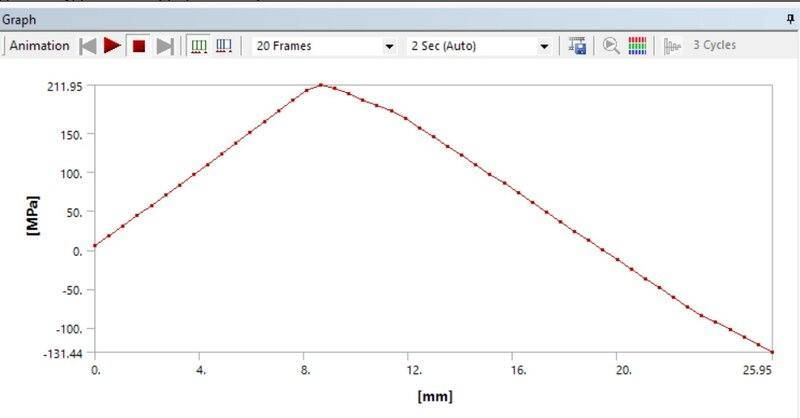Which matches very well with the first plot I have inserted here!

Also, the max stress before removing the bending moment, matches very well the calculation. I got ##\sigma_max = 457.8\mbox{ MPa}##, and from ANSYS, during the bending process we see that the max value is similar: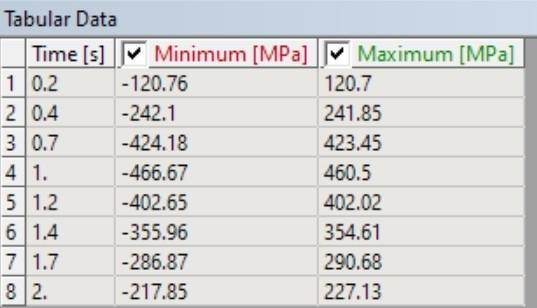Last edited:
freddie_mclair
Here are some images with the setup in ANSYS:

Material properties list: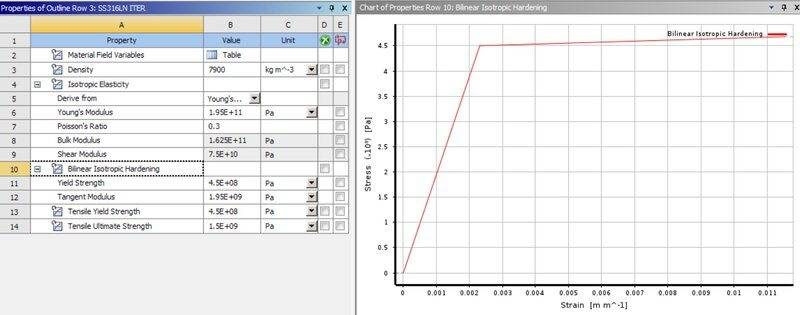Overall setup of the beam with the bending moment of 12.314kNm applied on both ends: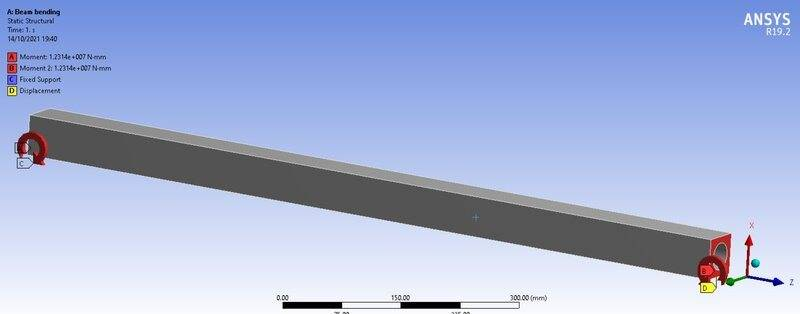Fixed edge on one end: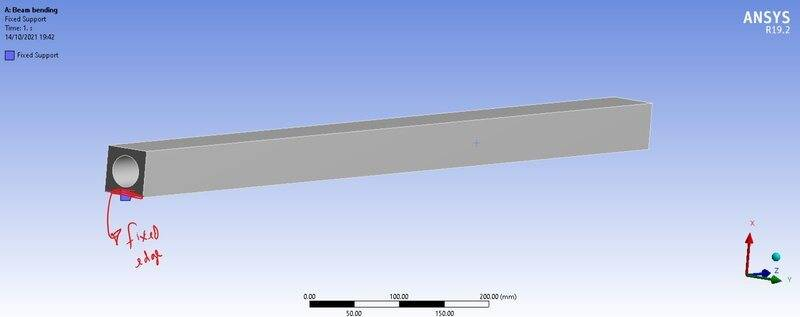Fixed displacement in xx on the other edge: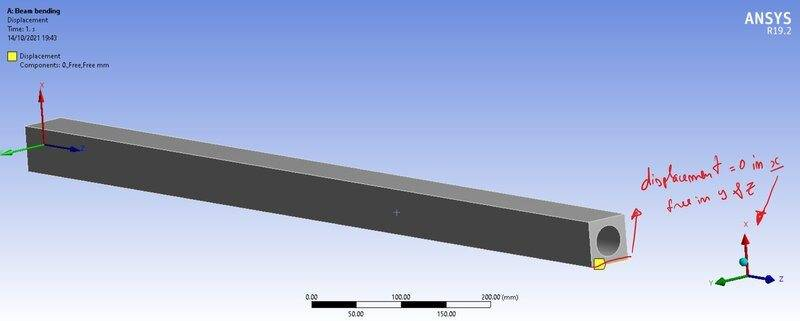#### Attachments

Last edited:
Thanks guys for your reply, but I'm looking for an analytical solution. The first thing I did was to compute what is the necessary bending moment, ##M_b## to get the radius I need, ##R_k## by computing the integral in Mathematica.
Then, with ##M_b## I can also get the stress profile in the cross-section by using ##\sigma_b = \frac{M_b}{J}x##.
But then, what do I have to subtract from ##\sigma_b## to get the residual stress after unloading?
Hi,
I am running into the same problem. I have a steel tube or let's say 36" steel pipe (hollow inside) and trying to figure out the residual stress after bending the pipe to a radius of 1600 feet. Are there any ready-made equations for residual stress on this type of cross-section? I saw some equations in other literature for rectangular elasto plastic bending and residual stress. Any insights on which books, or references I can use? Thank you

TL;DR Summary: After being a beam is bent, due to the springback effect, the resulting bent radius is larger than the bending radius. How can I estimate the residual stress that remains in the beam after an elasto-plastic loading/bending?

Hello everyone, I hope you can give me hand with what I will present.

I have a beam with cross-section shown below.

View attachment 287743
I considered a simple linear hardening model for predicting the stress and strain developed by bending the beam on the radius ##R_0## around the y-axis, where:

The strain, ##\varepsilon_L(x)##, can be defined as:
##\varepsilon_L(x) = \frac{x}{R_0}##

And the stress, ##\sigma_L(x)##, developed in the beam due to the loading by bending is:
##\sigma_L(x) = \begin{cases}
\frac{x}{R_0} \cdot E \mbox{, if } x \leq \varepsilon_y \cdot R_0\\
\sigma_L(x) = S_y+(\frac{x}{R_0}-\varepsilon_y)\cdot E_1\mbox{, if } x \gt \varepsilon_y \cdot R_0
\end{cases}##

Where and ##\varepsilon_y = \frac{S_y}{E}##, ##S_y## is the yield strength and ##E## the Young's Modulus.
##E_1## is the tangent modulus which is defined as shown in the picture below.

View attachment 287744

I also know that, due to the springback effect, the relationship between the bending radius ##R_0## and the resulting radius ##R_r## is given by:
##\frac{1}{R_0} - \frac{1}{R_r} = \frac{M_b}{EJ}##

Where ##M_b## is the bending moment and ##J## the moment of inertia (in this case ##J=\frac{h^4}{12}-\pi \frac{r^4}{4}##).

The bending moment can be estimated by:
##M_b=2\int_0^{h/2} \sigma_L(x) b(x) x dx##

Where ##b(x)\cdot x## is the cross-sectional area of the beam and ##b(x)## is defined by:
##b(x) = \begin{cases}
h-2\sqrt{r^2-x^2} \mbox{, if } x \leq r\\
h \mbox{, if } x \gt r
\end{cases}##

Now, with all this at hand, how can I estimate the residual stress on the beam after bending, i.e., after unloading the beam?

Hi, I am trying to run calcs for residual stress on a 36" steel pipe bent to a tight radius. Where do I find the equations and concepts you have used above? Any book? Are there ready-to-use equations to calculate residual stress for a steel pipe (since the shape is pretty typical). Any info is highly appreciated.

freddie_mclair
Hi, I am trying to run calcs for residual stress on a 36" steel pipe bent to a tight radius. Where do I find the equations and concepts you have used above? Any book? Are there ready-to-use equations to calculate residual stress for a steel pipe (since the shape is pretty typical). Any info is highly appreciated.
Hi, try to use the same method I described above. You just need to adapt the cross-sectional geometry of your beam, i.e., redefine ##b(x)## for a pipe and, of course, the mechanical properties of the material. Then apply the steps I've defined in this post.

freddie_mclair
Hi, I am trying to run calcs for residual stress on a 36" steel pipe bent to a tight radius. Where do I find the equations and concepts you have used above? Any book? Are there ready-to-use equations to calculate residual stress for a steel pipe (since the shape is pretty typical). Any info is highly appreciated.
For a pipe, you can define ##b(x)=\sqrt{(R_o^2-R_i^2)-x^2}##, where ##R_o## is the outer radius and ##R_i## the inner radius.

•